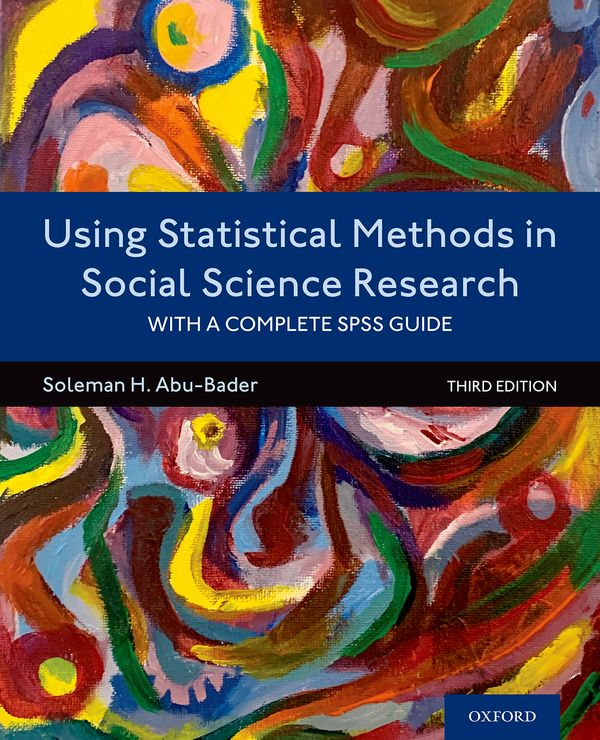Abu-Bader, Using Statistical Methods in Social Science Research, 3eDescription

Using Statistical Methods in Social Science Research, Third Edition is the user-friendly text every student needs for analyzing and making sense of quantitative data. With over 20 years of experience teaching statistics, Soleman H. Abu-Bader provides an accessible, step-by-step description of the process needed to organize data, choose a test or statistical technique, analyze, interpret, and report research findings.

The book begins with an overview of research and statistical terms, followed by an explanation of basic descriptive statistics. It then focuses on the purpose, rationale, and assumptions made by each test, such as Pearson's correlation, student's t-tests, analysis of variances, and simple linear regression, among others. The book also provides a wealth of research examples that clearly display the applicability and function of these tests in real-world practice. In a separate appendix, the author provides a step-by-step process for calculating each test for those who still like to understand the mathematical formulas behind these processes.

Resources for Using Statistical Methods in Social Science Research, 3e